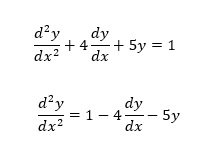# Solving Differential equations with Simulink: tutorial 2

In this tutorial, I will explain the working of differential equations and how to solve a differential equation. At the start a brief and comprehensive introduction to differential equations is provided and along with the introduction a small talk about solving the differential equations is also provided. After that a brief introduction and the use of the integral block present in the simulink library browser is provided and how it can help to solve the differential equation is also discussed. After that an example is performed in which a second order differential equation is solved on simulink along with the description of each step and the use and working of each block. At the end a simple exercise is provided regarding the concepts and blocks used in this tutorial.

### How to solve differential equations in simulink

Figure 1: Integration

• As the name suggests, this block is used to calculate the integral of the signal provided at the input i.e. left side of the block. In case of solving a differential equation, the major this we have to do is to integrate the given equation which will return the function without the derivative as is obvious from the equation below,
• Integration of the derivative of a function is equal to the function itself. The purpose of the integral block here is the same. Number of integral blocks used in a block diagram is equal to the order of the differential equation we are going to solve hereby in the problem. For instance, if we want to solve a 1st order differential equation we will be needing 1 integral block and if the equation is a 2nd order differential equation the number of blocks used is two. Lets’ now do a simple example using simulink in which we will solve a second order differential equation.

### Block diagram of differential equations in Simulink

Figure 2: Library browser

Figure 3: Commonly used blocks

• We will be writing a simulink program or in simple words we will create a block diagram that will solve the differential equation given below. The first step to be done here is to rearrange the differential equation. On the left hand side write the highest order derivative and move all the remaining terms on the right hand side as given in the equation below,Figure 4: Integral block

Figure 5: Second order integral

Figure 6: 3rd order integral

Figure 7: Gain block

Figure 8: Constant block

Figure 9: Sum block

Figure 10: Placed components

Figure 11: Flipping block

Figure 12: Changing gain

Figure 13: Gain blocks

Figure 14: Shape of sum block

Figure 15: List of signs

Figure 16: Constant

Figure 17: Derivation steps

Figure 18: Second term

Figure 19: Third term

Figure 20: Scope

Figure 21: Complete block diagram

Figure 22: Running the block diagram

Figure 23: Output

Exercise:

### 5 thoughts on “Solving Differential equations with Simulink: tutorial 2”

1. Please I would like To Built Digital Video Broadcast Using Auduino, For Transmitting and Receiving Like Now Days Digital Decorders, Now Will i Able to communicate with Arduino to work as Decorder?

2. (d^2 y)/(dt^2 )= dy/dt+y^2+3 with the initial conditions y(0)=1,(dy/dt)_0=-1
Using SIMULINK obtain the profiles of y(t),dy/dt, and (d^2 y)/(dt^2 ) over a simulated time period of 20 secs.